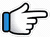# Lag Ja Gale Ke Phir Ye – Keyboard Notes## Lag Ja Gale Ke Phir Ye Keyboard Notes

For Lag Ja Gale Ke Phir Ye Notes as Western or Indian Notations, Click –

Western – CDE FGAB

Indian – srg mpdn

Hindi – सारेग मपधनि

## Keyboard Notes for Lag Ja Gale Ke Phir Ye – Indian Notation (srg mpdn)

CDE FGAB

सारेग मपधनि

### Scale and notations for Lag Ja Gale

Scale used for the song Lag Ja Gale is G major

Notes in G scale are –
p d n s r g M p

So the notes used for the song are –
r g M p d n s* r*

## 1. Simplified Keyboard Notes for Lag Ja Gale

Starting Music

np  gmp  g  srg s *n~

This can be played in higher octave –

n*p*  g*m*p*  g*  s*r*g* s* n~

### Part 1 – 1

Lag jaa gale
ke phir yeh

Haseen raat
ho na ho

r~      n~~   dn~~~
Lag   ja      gale

n       dn       n
ke     phir     ye
Or
n      dn       dn

ndn         dn~ p
haseen_ raat

pds*~   n     d~~  pMgr
ho~     na    ho~~
Or
pds*    n    d~ ndpgr

### Part 1 – 2

janam mein

Mulaqaat ho na ho

r~ g~         g        gg~

MdMg    r
janam    mein

rr r*~ r*g*      s*r*~
Mulaa_kat     ho

s*       n~ dp
naa    ho~~

### Repeat Part 1

r~     n~~  dn~~~  n   dn   n
Lag  ja     gale     ke  phir  ye

ndn          dn~ p  pds*~   n    d~~  pMgr
haseen_ raat     ho~      na  ho~~

r~ g~         g     gg~  MdMg  r

rr r*~ r*g*  s*r*~    s*     n~ dp
Mulaa_kat       ho        naa    ho~~

r        n      dn    n  n   n
Lag   jaa  gale  se e~e~

### Music

Music Part 1

pMrgpM

rg gggg
gM MMMM
Mp pppp

(How to play –
r then g 5 times,
g then M 5 times
M then p 5 times)

Music Part 2

Repeat:

pMrgpM

rg gggg
gM MMMM
Mp ppp

Music Part 3

rgpng*r*npdM
(Or rpng*r*npdM)
rgMgr
gMr
gMr
gMr

### Part 2 – 1

Humko mili hain aaj yeh

p~       r*    R*r*   r*
Hum   ko   mili    hai

R*r*g*r*    s*   s*
aa~~        j_   ye
Or
r*g*r*      s*   s*
g*r*s*      s*    s*

ps*s*         g*r*s*r*s*n    n
Or
ps*s*       g*s*r*s*    n   n

Music

nr*nr*~  ds*ds*~  pnpn~

Repeat:

p~       r*    R*r*   r*
Hum    ko  mili     hai

R*r*g*r*  s*   s*
aa~~      j_   ye

ps*s*         g*r*s*r*s*n    n
Or
ps*s*         g*s*r*s*n    n

### Part 2 – 2

Jee bhar ke dekh leejiye
Humko qareeb se

p          r*~      R*
Jee     bhar     ke

r*~ r*    M*g*r*~ s*s*
dekh    lee__     jiye

ps*s*
Hum_ko

g*r*s*r*s*  n     n
karee~~     b_   se
Or
g*r*s*r*s*         n
g*r*s* s*r*s*n   n

### Part 3

Phir aap ke naseeb mein
Yeh baat ho na ho

Mulaqaat ho na ho

r         n~ dn
Phir   aa_pke

ndndn     n      n
nasee~~ b     mein

n       dnp
Ye     baat

pds*   n       dpMg
ho     na     ho

r~ g~        g     gg~

MdMg     r
janam    mein

rr r*~ r*g*
Mulaa_kat

s*r*~    s*     n~ dp
ho      naa    ho~~

r         n      dn    n  n   n
Lag   jaa  gale  se e~e~

### Music

(same as previous music notes)

pMrgpM

rg gggg
gM MMMM
Mp pppp

(How to play –
r then g 5 times,
g then M 5 times
M then p 5 times)

pMrgpM

rg gggg
gM MMMM
Mp ppp

rgpng*r*npdM
(Or rpng*r*npdM)
rgMgr
gMr
gMr
gMr

### Part 4

Notes are similar to part 2

Paas aaiye ke hum nahi,
aayenge baar baar

Bahen gale mein daal ke
Hum ro-le zaar zaar

p~        r*~ R*r*   r*
Paas   aaiye      ke

R*r*g*r*  s*   s*
hum~~    nahi,
Or
r*g*r*       s*   s*
g*r*s*     s*    s*

ps*s*     g*   r*s*r*s*n n
aayen   ge  baar     baar
Or
ps*s*    g*  s*r*s*n n

p~  r*      R*r*   r*
Bahen   gale    mein

R*r*g*r*  s*   s*
daa~~   l    ke

ps*     s* g*   r*s*r*s*n n
Hum  ro-le    zaar zaar
Or
ps*    s* g*  s*r*s*n  n

### Part 5

Ankhoon se phir ye
pyaar ki barsaat ho naa ho

Mulakaat ho na ho

r    n~      d  n     n
Ankhoon se phir ye

dn  n  n    dnnp
pyaar ki  barsaat

pds*   n      dpMg
ho     na     ho

r~ g~         g     gg~  MdMg  r

rr r*~ r*g*     s*r*~    s*     n~ dp
Mulaa_kat   ho        naa    ho~~

## 2. Full Keyboard Notes For Lag Ja Gale

Starting Music

np  gmp  g  srg s *n~

Part 1

r~  n~~  dn~~~  n  dn  n  |  ndn  dn~ p  pds*~  n  d~~  pMgr
Lag jaa gale ke phir yeh  |  Haseen raat ho na ho

r~ g~  g  gg~  MdMg  r  |  rr r*~ r*g*  s*r*~  s*  n~ dp
Shaayad phir iss janam mein  |  Mulaqaat ho na ho

Repeat Part 1

r~ n~~ dn~~~ n dn n | ndn dn~ p pds*~ n d~~ pMgr
Lag jaa gale ke phir yeh | Haseen raat ho na ho

r~ g~ g gg~ MdMg r | rr r*~ r*g* s*r*~ s* n~ dp
Shaayad phir iss janam mein | Mulaqaat ho na ho

r  n  dn  n  n  n
Lag  jaa  gale  se e~e~

Music

pMrgpM  |  rg gggg  gM MMMM  Mp pppp

pMrgpM  |  rg gggg  gM MMMM  Mp ppp

rgpng*r*npdM  |  rgMgr  gMr  gMr  gMr

Part 2

p~  r*  R*r*  r*  R*r*g*r*  s*  s*  |  ps*s*  g*r*s*r*s*n  n
Humko mili hain aaj yeh |  Ghadiyaan naseeb se

Music

nr*nr*~  ds*ds*~  pnpn~

Repeat:

p~  r*  R*r*  r*  R*r*g*r*  s*  s*  |  ps*s*  g*r*s*r*s*n  n
Humko mili hain aaj yeh |  Ghadiyaan naseeb se

p  r*~  R*  r*~ r*  M*g*r*~ s*s*  |  ps*s*  g*r*s*r*s* n  n
Jee bhar ke dekh leejiye  |   Humko qareeb se

Part 3

r  n~ dn  ndndn  n  n  |  n  dnp  pds*  n  dpMg
Phir aap ke naseeb mein  |  Yeh baat ho na ho

r~ g~  g  gg~  MdMg  r  |  rr r*~ r*g*  s*r*~  s*  n~ dp
Shayad phir is janam mein  |  Mulaqaat ho na ho

r  n  dn  n  n  n
Lag  jaa  gale  se e~e~

Part 4

p~  r*~ R*r*  r*  R*r*g*r*  s*  s*  |  ps*s*  g*  r*s*r*s*n n
Paas aaiye ke hum nahi  |  aayenge baar baar

p~  r*  R*r*  r*  R*r*g*r*  s*  s*  |  ps*  s* g*  r*s*r*s*n n
Bahen gale mein daal ke  |  Hum ro-le zaar zaar

### Part 5

r  n~  d  n  n    |  dn  n  n  dnnp pds*  n  dpMg
Ankhoon se phir ye  |  pyaar ki barsaat ho naa ho

r~ g~  g  gg~  MdMg  r  |  rr r*~ r*g*  s*r*~  s*  n~ dp
Shayad phir is janam mein  |  Mulakaat ho na ho

Song List

Bhajan List

New Song List